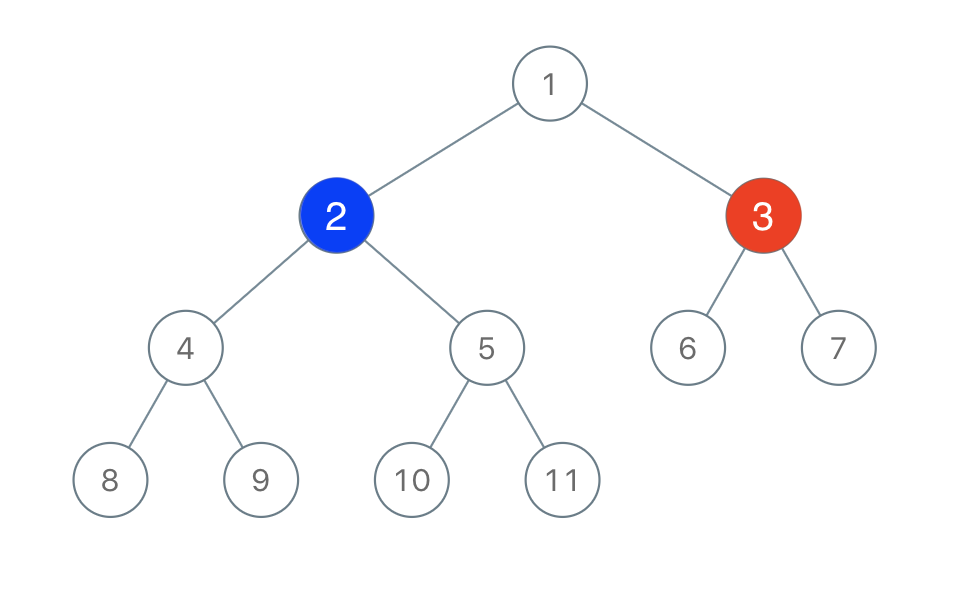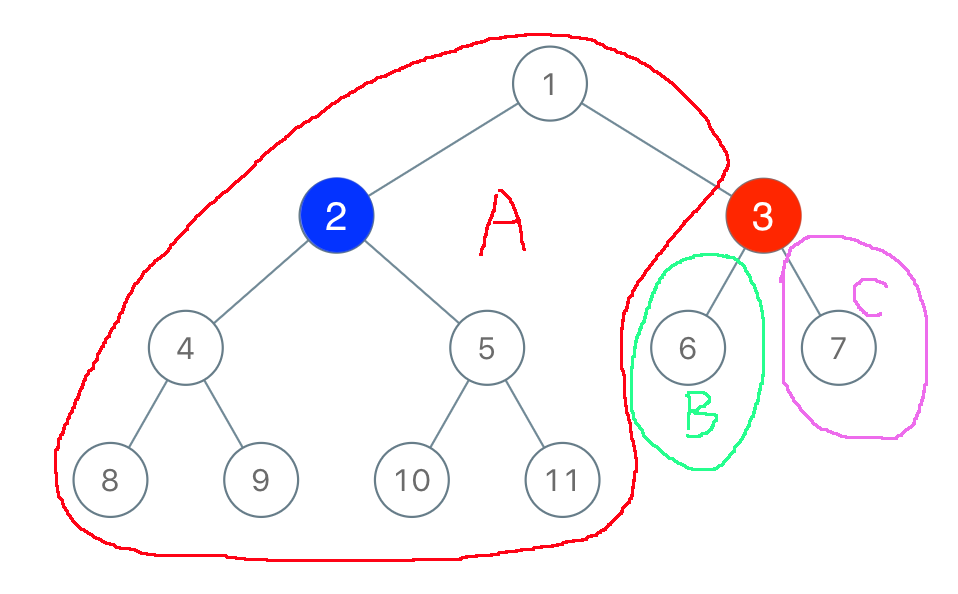Leetcode 1145：二叉树着色游戏（超详细的解法！！！）
in leetcode with 0 comment

「一号」玩家从 [1, n] 中取一个值 x1 <= x <= n）；

「二号」玩家也从 [1, n] 中取一个值 y1 <= y <= n）且 y != x

「一号」玩家给值为 x 的节点染上红色，而「二号」玩家给值为 y 的节点染上蓝色。输入：root = [1,2,3,4,5,6,7,8,9,10,11], n = 11, x = 3

• 二叉树的根节点为 root，树上由 n 个节点，节点上的值从 1n 各不相同。
• n 为奇数。
• 1 <= x <= n <= 100class Solution:
def btreeGameWinningMove(self, root: TreeNode, n: int, x: int) -> bool:
c = [0, 0]
def helper(node):
if not node:
return 0
l, r = helper(node.left), helper(node.right)
if node.val == x:
c, c = l, r
return l + r + 1
return helper(root) // 2 < max(max(c), n - sum(c) - 1)

reference:

https://leetcode.com/problems/binary-tree-coloring-game/discuss/350570/JavaC%2B%2BPython-Simple-recursion-and-Follow-Up

「如果我的文章对你有很大帮助，那么不妨～！」Responses